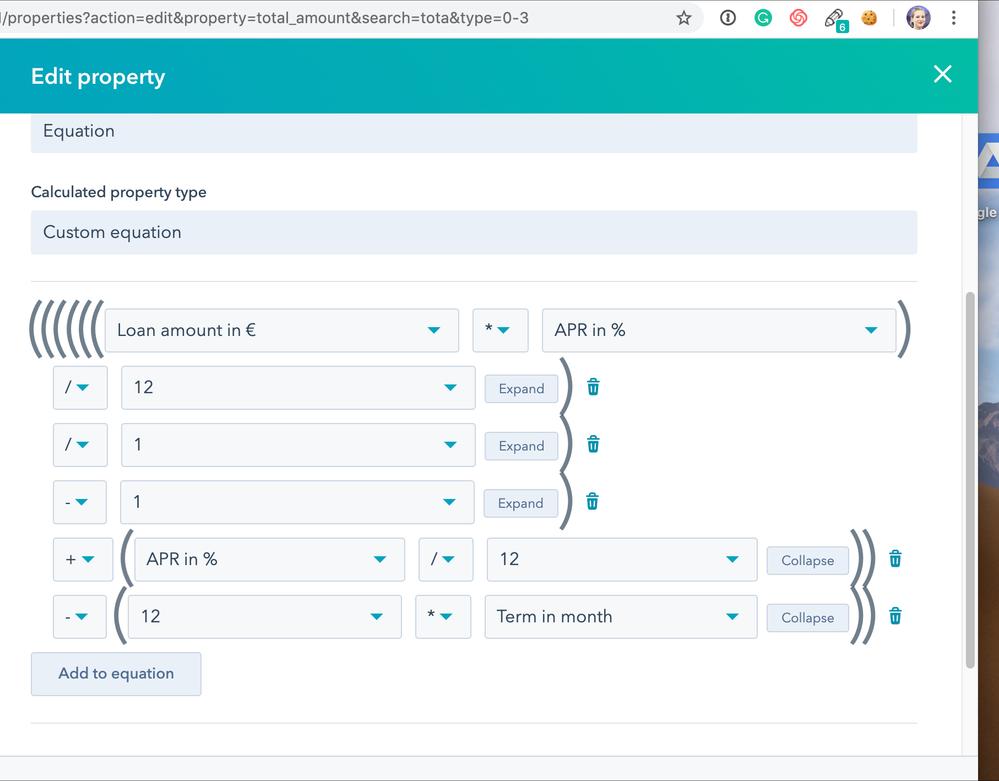## CRMParticipant

## Hubspot calculation property - Function power/exponent

Hi Hubspot community,

As we are a bank, we need to calculate interest rates. I created a new property, however, I need the power function / exponent for my calculation.

Here is my function: [Loan amount × APR/12]/[1−(1 + APR/12)^−Term

Hubspot told me that was not possible. Do you have an idea to manage this problem or handle this limit?

Thank you so much,

2 Replies 2## Hubspot calculation property - Function power/exponent

Hi @PriscillaMB

Can try using custom javascript, using https://developers.hubspot.com/manipulating-forms-with-jquery

Thanks!
AjitParticipant

## Hubspot calculation property - Function power/exponent

I am not sure to understand the link you send me.

I create a new property on the deal (not on a form) to calculate the monthly payment for a loan. The calculation is: [Loan amount × APR/12]/[1−(1 + APR/12)^−Term

Loan amount, APR (rate in %) and Term are other custom properties I created on the deal.

However I don't reach to set up my formula because of the system limit, I can not use an exponential function

ThanksTicket Hubspot - Calculation property